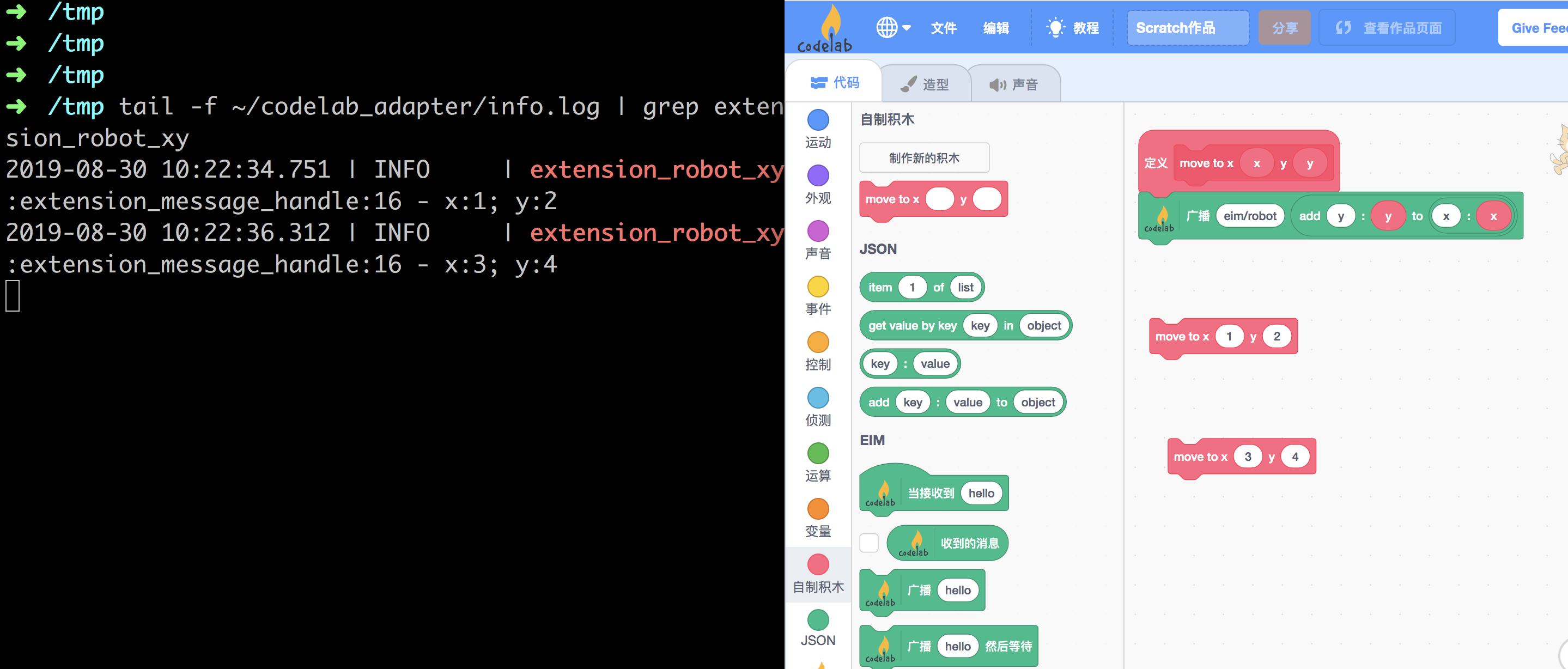# json message¶

hello world 案例中，我们构建了这样一个程序：接收一个来自 Scratch3 的字符串（`hello world`），将其反转后（`dlrow olleh`）返回。

2. 使用 Scratch3 EIM extension，传递 json 消息。

## 传递 x、y 坐标¶

1. 在 Scratch3 中自定义新的积木
• 使用 Scratch3 json extension 构建 json 数据
• 使用 Scratch3 EIM extension 传递消息到 CodeLab Adapter
2. 构建 CodeLab Adapter 插件：`extension_robot_xy.py`，在插件中处理传递过来的`(x,y)`

## 在 Scratch3 中自定义新的积木¶``````import time

class RobotXYExtension(Extension):
NODE_ID = "eim/robot"
s
def __init__(self):
super().__init__()

if type(content) == dict:
x = content["x"]
y = content["y"]
self.logger.info(f'x:{x}; y:{y}')

def run(self):
while self._running:
time.sleep(1)

export = RobotXYExtension
``````

## 开始测试¶## json message from CodeLab Adapter to Scratch3¶

``````import time
import random

class RobotXYExtension(Extension):
NODE_ID = "eim/robot"

def __init__(self):
super().__init__()

if type(content) == dict:
x = content["x"]
y = content["y"]
self.logger.info(f'x:{x}; y:{y}')

def run(self):
while self._running:
message = self.message_template()
random_x = random.randint(-240,240)
random_y = random.randint(-180,180)
self.publish(message)
time.sleep(1)

export = RobotXYExtension
``````## 提醒¶

Scratch 内部的消息就只支持字符串，这是一种很好的设计，因为它简单易理解。